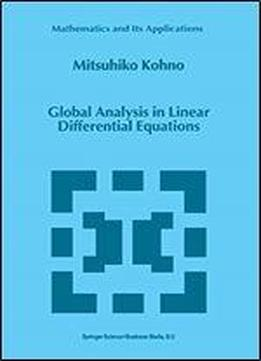# Global Analysis In Linear Differential Equations (mathematics And Its Applications) by M. Kohno / 2012 / English / PDF

Since the initiative works for global analysis of linear differential equations by G.G. Stokes and B. Riemann in 1857, the Airy function and the Gauss hypergeometric function became the most important and the greatest practical special functions, which have a variety of applications to mathematical science, physics and engineering. The cffcctivity of these functions is essentially due to their "behavior in the large" . For instance, the Airy function plays a basic role in the asymptotic analysis of many functions arising as solutions of differential equations in several problems of applied math ematics. In case of the employment of its behavior, one should always pay attention to the Stokes phenomenon. On the other hand, as is well-known, the Gauss hypergeometric function arises in all fields of mathematics, e.g., in number theory, in the theory of groups and in analysis itself. It is not too much to say that all power series are special or extended cases of the hypergeometric series. For the full use of its properties, one needs connection formulas or contiguous relations.

views: 251